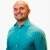# Graph an absolute value inequality from the parent graphThis lesson will look at an example problem involving absolute value inequalities. This lesson will give an explanation of how to graph an absolute value inequality from the parent graph with a step by step look... This lesson will look at an example problem involving absolute value inequalities. This lesson will give an explanation of how to graph an absolute value inequality from the parent graph with a step by step look to better explain the concept.
More... Collapse